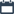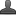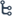## Tuesday, October 13, 2020

•October 13, 2020
•••1 comment

## Andhra pradesh SSC Mathematics Trigonometry Do This & Try this Solutions Class 10th

Mathematics trigonometry DO THIS & TRY THIS Sums Solutions

Hi friends and my dear students! In this post, I have covered Andhra Pradesh SSC(10th class) Mathematics trigonometry Do This & Try this Solutions. After Reading Mathematics trigonometry Exercise, Please do share it with your friends. Learn Maths for All Classes here.

DO THIS

1. Identify  "Hypotenuse", "Opposite side" and "Adjacent side" for the given triangles

Sol.  ln the triangle PQR

Opposite side =PQ

Hypotenuse = PR

2.1) for angle X

2) For angle Y

Sol. In the triangle XYZ

1)     För angle X

Opposite side = YZ

Hypotenuse= XY

2. For angle Y

Opposite side =XZ

Hypotenuse = XY

Also Check

Introduction To Trigonometry

Introduction to Knowing Our Numbers Key Points

SSC (10th class) Trigonometry Exercise - 11.1 Solution

SSC(10th class) Trigonometry Exercise - 11.1 Solutions

3. In triangle XYZ, angle Y is right angle, XY=17 cm and YZ=15 cm, then find (i) sin X (ii) cos Z.  (iil )tanX

Sol:   Given  triangle XYZ,

Y is right angle.

By Pythagoras theorem

XZ  = YZ 2 +XY 2

XY 2 = 172-152

XY 2 = 289-225

XY 2 = 64

XY = √64

XY = 8

i)  sin X =  opposite side to x / Hypotenuse

=  YZ / XZ=15/17

ii) cos Z = Adjacent side to x / Hypotenuse

= YZ / XZ=15/17

iii) tan X = Opposite side to X/ Adjacent side to X

= YZ/XZ=15/ 8

TRY THIS:

1. Write length of "Hypotenuse", "Opposite side" and "Adjacent side" for the given angles in the given triangles.

i)  For angle C

ii)  For angle A

Sol.     By Pythagoras theorem

AC 2 = AB 2 + BC 2

(5)2 = AB 2 +42

25 =AB2+16

AB 2 = 25 –16

AB 2 =9

AB = V 9

AB    = 3

For angle C:

Opposite side = AB = 3 cm

Adjacent side = BC = 4 cm

Hypotenuse = AC = 5 cm

For angle A:

Opposite side = BC = 4 cm

Adjacent side =AB = 3 cm

Hypotenuse AC = 5 cm

2. In a right angle triangle ABC, right angle is at C. BC + CA = 23 cm and BC- CA = 7cm  than find sin A and tan B

Sol. In a right angle triangle ABC, right angle is at C.

Given that

BC+CA = 23……... (1)

BC-CA =7………. .(2)

Solve equation 1 and 2

BC+CA = 23……... (1)

BC-CA =7………. .(2)

................................

BC = 30/2

BC =15

Substituting BC =15 in equation (1)

BC + CA = 23

CA = 23 – BC

CA = 23 - 15

CA = 8

By Pythagoras theorem

AB 2 = AC 2 + BC 2

= 82 +152

= 64+22

AB 2= 289

AB =√ 289

=√17 X 17

=17

sin A =BC/AB = 15/17

tan B = AC/BC= 8/ 15

#### 1 comment:

1.You are sharing a particularly decent article here. It is a significant and factual article for us. Thankful to you for sharing an article like this.abacus maths classes